# Chapter 2.1. Simple Calculations

In this chapter, we are going to get familiar with the following concepts and programming techniques:

• What is system console?
• How to read numbers from the system console?
• How to work with data types and variables, which are necessary to process numbers and the operations between them?
• How to print (a number) on the console?
• How to do simple arithmetic operations: add, subtract, multiply, divide, string concatenation?

## Video

Watch a video lesson on this chapter here: https://www.youtube.com/watch?v=7u4X5X-a9Qg.

## The system console

The system console or the computer console, usually called just a console, represents the tool by which we give the computer commands in a text format and get the results from their execution again as a text.

Generally, the system console represents a text terminal which means that it accepts and visualizes just text without any graphical elements like buttons, menus, etc. Usually, it looks like a black-colored window, in which we write and perform console commands and print the result in the form of a text. In Windows, the console looks like this: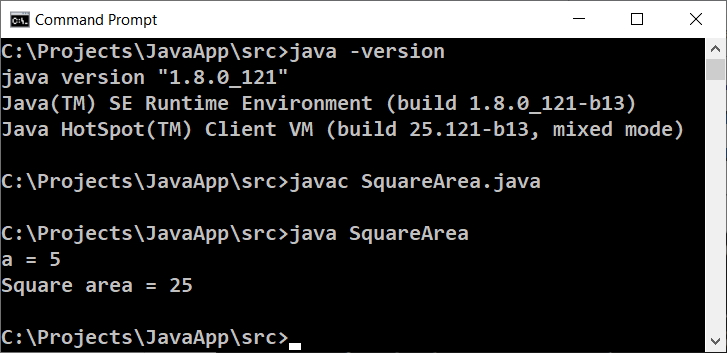In most operating systems, generally, the console is available as a standalone application on which we write different console commands. In Windows, it is called Command Prompt, while in Linux and macOS, it is called Terminal. The console runs console applications. They read the text as input from the command line and print the output as a text on the console. In this book, we are going to learn programming mostly through creating console applications.

This is how the console looks in Linux: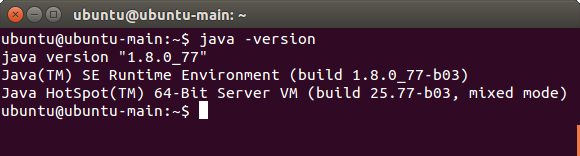## Reading integers from the console

In Java we have several options to read an input from the console. The one way is via the built-in class Scanner, which we will use now, and the other is via BufferedReader. The second one is a bit faster, but we will start using it after we get familiar with the streams in Java.

То read an integer (not floating-point number) from the console we have to import the built-in class Scanner on the first line of our program even before the base class declaration. We put this line at the top:

import java.util.Scanner;


Then in the main(…) method of the program, we have to create a new Scanner instance used to attach the system input. After that (via the created scanner reader) we can read integers, floating-point numbers, text, etc. For example, with .nextInt() we read the next integer given as input:

Scanner scanner = new Scanner(System.in);
int num = scanner.nextInt();


In the example above, in the variable num of integer type int, we will have a value read as input from the console.

Java is highly typed language this means that the variables have type determine at compile-time and can not change at the execution time (as it is in dynamic languages such as JavaScript and Python). In the example above scanner is from type java.util.Scanner (scanning text reader) and the variable num is from type int (integer number).

### Example: Calculating the area of the square with side а

As an example, let us look at a program. The task is to calculate the area of a square by a given side's length read as input from the console. The sample source code of the program is below. The code reads an integer as input from the console, multiply it by itself (squares it), and as output prints the result from the multiplication. Save the code in a file with the name SquareArea.java, or else you will have a compile-time error:

// Put this code in the file: SquareArea.java

import java.util.Scanner;

public class SquareArea {
public static void main(String[] args) {
Scanner scanner = new Scanner(System.in);

System.out.print("a = ");
int a = scanner.nextInt();
int area = a * a;

System.out.print("Square area = ");
System.out.println(area);
}
}


Here is how the program would work when we have a square with a side's length equal to 15: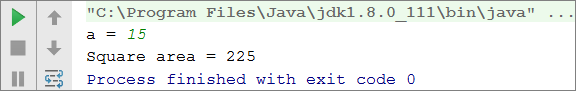Test the program with an invalid integer (as an input), for example, "hello". You will get an error message during runtime (exception).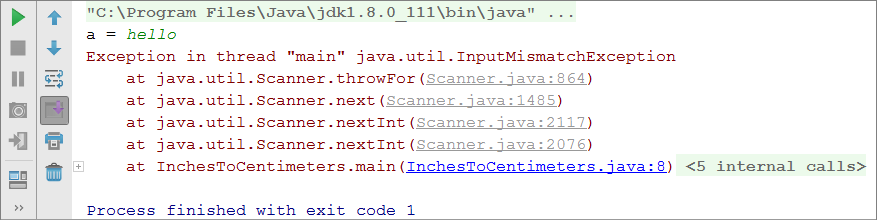It is normal because Java is a highly typed language. Later on, we will find out how we can catch these kinds of errors and make the user enter a new input (a number in the example above).

#### How Does the Example Work?

The first line Scanner scanner = new Scanner(System.in); creates a new instance from the Scanner class with standard system input.

The following line System.out.print("a = "); prints an informative message which invites the user to enter the side a of the square. After the output is printed the cursor stays on the same line. Staying on the same line is more convenient for the user visually. We use System.out.print(…); and not System.out.println(…); and this way the cursor stays on the same line.

The following line int a = scanner.nextInt(); reads an integer from the console. The result is kept in a variable with a name a.

The following line int area = a * a; keeps the result of the multiplication of a by a in a new variable with a name area.

The following line System.out.print("Square area = "); prints the given text without going to the next line. Again, use System.out.print(…); and not System.out.println("…"); and this way the cursor stays on the same line to print the calculated area of the square afterward.

At the last line System.out.println(area); prints the calculated value of the variable area.

#### Reading floating-point numbers from the console

Try to rewrite the above program to accept as input data not only integers (int) but floating-point numbers (double). You can use scanner.nextDouble(). Later in this chapter, we will learn more about floating-point numbers.

## Calculations in programming

We know about computers that they are machines that process data. All data is stored in the computer memory (Random-access memory(RAM)) in variables. A variable is the name of a reserved area allocated in memory that stores data from certain types, for example, numbers or text. Each variable in Java has name, type and value. In the example below, you can see how to define a variable, and at the same time to assign value to it: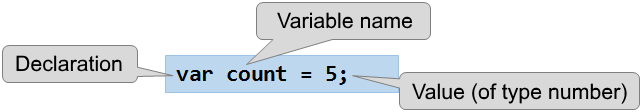After processing, the data is stored again in variables (somewhere in a reserved area allocated in memory by our program).

## Data types and variables

In programming, each variable stores a certain value of a particular type. For example, data types can be: number, letter, text (string), date, colour, image, list and others. Here are some examples of data types:

• type int number: 1, 2, 3, 4, 5, …
• type double number: 0.5, 3.14, -1.5, …
• type a letter from the alphabet (symbol): 'a', 'b', 'c', …
• type text (string): "Hello", "Hi", "Beer", …
• type day of the week: Monday, Tuesday, …

## Reading floating-point numbers from the console

To read floating-point number from the console, again it is neccessary to declare variable, to point out the type of the number, and to use the standard command to read information from the system console:

Scanner scanner = new Scanner(System.in);

Double num = scanner.nextDouble();


### Example: Converting inches into centimeters

Write a program that reads a floating-point number (representing inches) as an input from the console, converts it to centimeters, and print the result as output:

Scanner scanner = new Scanner(System.in);

System.out.print("Inches = ");
Double inches = scanner.nextDouble();
Double centimeters = inches * 2.54;
System.out.print("Centimeters = ");
System.out.println(centimeters);


Run the program and make sure that when entering a value in inches, we obtain a correct output in centimeters: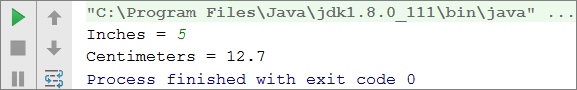#### Testing in the Judge system

To read a text (string) from the console, again we declare new variable and use the standard command for reading a text from the console:

Scanner scanner = new Scanner(System.in);

String str = scanner.nextLine();


Let's pay attention to the fact that reading text does not require converting to another type. It is this way because by default, the method scanner.nextLine(…) returns as result text. Additionally, we can parse the text to an integer by Integer.parseInt(…) or floating-point number by Double.parseDouble(…). If we do not parse to a number, each number will be just text for the program, and we can not do arithmetic operations with them.

### Example: Greeting by name

Write a program that reads the user's name as input from the console and greets him with the text Hello, name, where name is the name read as input data.

Scanner scanner = new Scanner(System.in);

String name = scanner.nextLine();
System.out.printf("Hello, %s!", name);


In this case the expression %s is replaced with the first passed argument, which is the variable name: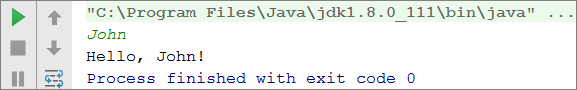## Concatenating text and numbers

When printing a text, numbers and other data on the console we can join them by using templates %s, %d, %f, etc. In programming, these templates are called placeholders. Depending on the type of the variable, via different symbols we declare different types of arguments. The main ones are %s for text (string), %d for integers, %f for floating-point numbers.

Here is example for printing formatted text with (placeholders):

Scanner scanner = new Scanner(System.in);

String firstName = scanner.nextLine();
String lastName = scanner.nextLine();
int age = Integer.parseInt(scanner.nextLine());
String town = scanner.nextLine();
System.out.printf("You are %s %s, a %d-years old person from %s.",
firstName, lastName, age, town);


Here is the result, which we will get after the execution of the example: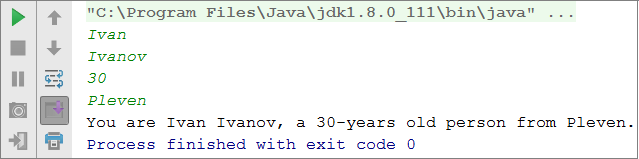Pay attention to the order of variables in the System.out.printf() method. Each variable is in the order we want to print it and corresponds to the type in the placeholder. Essentially, the template (placeholder) accepts variables of every type.

## Arithmetic operations

Let’s look at the basic arithmetic operations in programming.

### Summing up numbers (operator +)

We can sum up numbers using the operator +:

int a = 5;
int b = 7;
int sum = a + b; // the result is 12


### Subtracting numbers (оператор -)

Subtracting numbers is done by the operator -:

Scanner scanner = new Scanner(System.in);

int a = Integer.parseInt(scanner.nextLine());
int b = Integer.parseInt(scanner.nextLine());
int result = a - b;
System.out.println(result);


Here is the result of the execution of the program above (with numbers 10 and 3):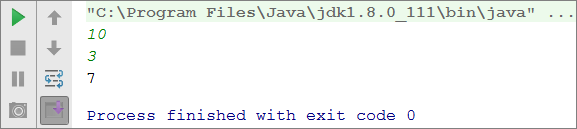### Multiplying numbers (operator *)

For multiplication of numbers we use the operator *:

int a = 5;
int b = 7;
int product = a * b; // 35


### Dividing numbers (operator /)

We divide numbers using the operator /. It works differently with integers and floating-point numbers.

• When we divide two integers, an integer division is applied, and the obtained output is without its fractional part. Example: 11 / 3 = 3.
• When we divide two numbers, and at least one of them is a floating-point number a fractional division is applied, and the obtained result is a floatting-point number, just like in math. Example 11 / 4.0 = 2.75. When division is not with exact precision, the result is being rounded, for example 11.0 / 3 = 3.66666666666667.
• The integer division by 0 causes an exception during runtime (Java.lang.ArithmeticException).
• Floating-point number divided by 0 do not cause an exception and the result is +/- infinity or the special value NaN. Example 5 / 0.0 = ∞.

Here are a few examples with the division operator:

int a = 25;
int i = a / 4;      // we perform the so-called integer division:
// the result of this operation will be 6 - the fractional part is cut off,
// as we perform division by integers
int f = a / 4.0;    // 6.25 - fractional division. We have explicitly specified that the number 4 should be interpreted as a fraction,
// adding the decimal point followed by zero
int error = a / 0;  // Error: integer division by 0


Let's look at a few examples for integer division (remember that when we divide integers in Java the result is an integer):

int a = 25;
System.out.println(a / 4);  // Integer result: 6
System.out.println(a / 0);  // Error: integer division by 0


Let's look at a few examples for floating numbers division. When we divide floating-point numbers, the result is always a float number and the division never fails and works correctly with the special values +∞ и -∞:

int a = 15;
System.out.println(a / 2.0);        // Fractional result: 7.5
System.out.println(a / 0.0);     // Result: Infinity
System.out.println(-a / 0.0);    // Result: -Infinity
System.out.println(0.0 / 0.0);   // Result: NaN (Not a Number), i.e. the result
// of the operation is not a valid numeric value


When printing the values and -∞ the console output may be ?, because the console in Windows does not work correctly with Unicode and breaks most of the non-standard symbols, letters and special characters. The example above would most probably give the following result:

7.5
?
-?
NaN


## Concatenating text and numbers

The operator + besides for summing up numbers, is also used for joining text (concatenation of two strings one after another). In programming, joining text with other text or with number is called "concatenation". Here is how we can concatenate a text with a number with the operator +:

String firstName = "Maria";
String lastName = "Ivanova";
int age = 19;
String str = firstName + " " + lastName + " @ " + age;
System.out.println(str);  // Maria Ivanova @ 19


Here is another example:

double a = 1.5;
double b = 2.5;
String sum = "The sum is: " + a + b;
System.out.println(sum);  // The sum is: 1.52.5


Did you notice something strange? Maybe you expected the numbers a and b to be summed? Actually, the concatenation works from left to right and the result above is absolutely correct. If we want to sum the numbers we have to use brackets in order to change the order of execution of the operations:

double a = 1.5;
double b = 2.5;
String sum = "The sum is: " + (a + b);
System.out.println(sum);  // The sum is: 4


## Numerical expressions

In programming, we can calculate numerical expressions, for example:

int expr = (3 + 5) * (4 – 2);


The standard rule for the precedence of arithmetic operations is applied: multiplying and dividing are always done before adding and subtracting. In the case of an expression in brackets, it is calculated first, but we already know all of that from math.

### Example: Calculating trapezoid area

Write a program that reads as input from the console the lengths of the two bases of a trapezoid and its height (one floating-point number per line) and calculates the area of the trapezoid by the standard math formula.

Scanner scanner = new Scanner(System.in);

double b1 = Double.parseDouble(scanner.nextLine());
double b2 = Double.parseDouble(scanner.nextLine());
double h = Double.parseDouble(scanner.nextLine());
double area = (b1 + b2) * h / 2.0;
System.out.println("Trapezoid area = " + area);


If we start the program and enter values for sides: 3, 4, and 5, we will obtain the following result:

3
4
5
Trapezoid area = 17.5


### Example: Circle area and perimeter

Write a program that reads as input from the console a radius r of a circle, and calculates its area and perimeter.

Formulas:

• Area = π * r * r
• Perimeter = 2 * π * r
• π ≈ 3.14159265358979323846…
Scanner scanner = new Scanner(System.in);

System.out.print("Enter circle radius. r = ");
double r = Double.parseDouble(scanner.nextLine());
System.out.println("Area = " + Math.PI * r * r);
// Math.PI - built-in function in Java
System.out.println("Perimeter = " + 2 * Math.PI * r);


Let’s test the program with radius r = 10: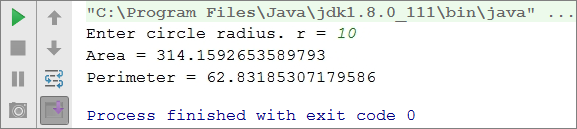### Example: 2D rectangle area

A rectangle is given with the coordinates of two of its opposite angles. Write a program that calculates its area and perimeter: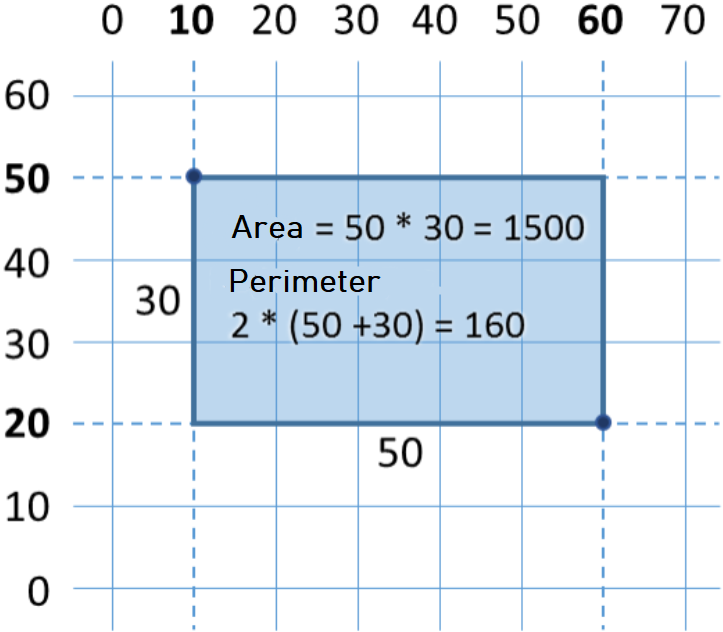In this tasк, we must take into account that we can obtain the length of the rectangle if we subtract the smaller x from the bigger x. Identically, if we subtract the smaller y from bigger y, we can obtain the rectangle height. Then we multiply both sides. Here is an example of an implementation of the described logic:

Scanner scanner = new Scanner(System.in);

double x1 = Double.parseDouble(scanner.nextLine());
double y1 = Double.parseDouble(scanner.nextLine());
double x2 = Double.parseDouble(scanner.nextLine());
double y2 = Double.parseDouble(scanner.nextLine());

// Calculate the sides of the rectangle:
double width = Math.max(x1, x2) - Math.min(x1, x2);
double height = Math.max(y1, y2) - Math.min(y1, y2);

DecimalFormat decimalFormat = new DecimalFormat("#.#########");
System.out.println(decimalFormat.format(width * height));
System.out.println(decimalFormat.format(2 * (width + height)));


We use Math.max(a, b) to find the bigger value from a and b and identically Math.min(a, b) to find the smaller of both values.

When the program is executed with the values from the coordinate system above we obtain the following result: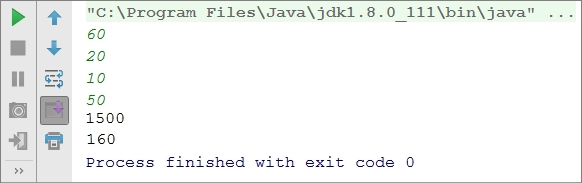## What we have learned in this chapter?

To summarize what have we learned in this chapter of the book:

• Reading a text: String str = scanner.nextLine(); (as we have written in advance Scanner scanner = new Scanner(System.in);).
• Reading an integer: int num = Integer.parseInt(scanner.nextLine());.
• Reading a floating-point number: double num = Double.parseDouble(scanner.nextLine());.
• Calculations with numbers and using the relevant arithmetic operators [+, -, *, /, (, )]: int sum = 5 + 3;.
• Printing a text by placeholders on the console: System.out.printf("%d + %d = %d", 3, 5, 3 + 5);.

## Exercises: Simple calculations

To consolidate our knowledge of simple calculations, let's solve several exercises.

### Blank IntelliJ IDEA solution (Project)

We start by creating an empty solution (Project) in IntelliJ IDEA. The solutions (project) in IntelliJ IDEA combine a group of tasks. This opportunity is very convenient when we want to work on a few projects and switch quickly between them or we want to consolidate logically a few interconnected projects.

In the current practical exercise we will use a Project with a couple of tasks (Java classes) to organize the solutions of the tasks from the exercises – every task in a separate Java class and all of them in a common project.

• We start IntelliJ IDEA.
• We create a new Project: [File] → [New] → [Project].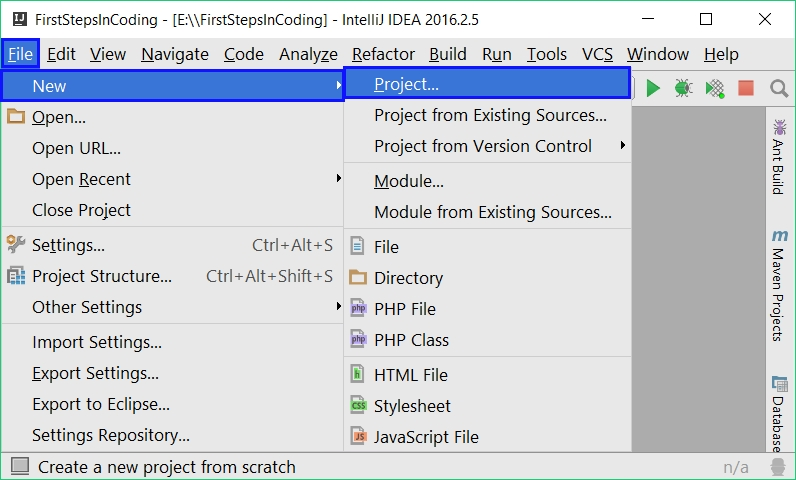Select from the dialog window [Java], in the field Project SDK we specify which version of Java we will use and finally we click [Next] → [Next]: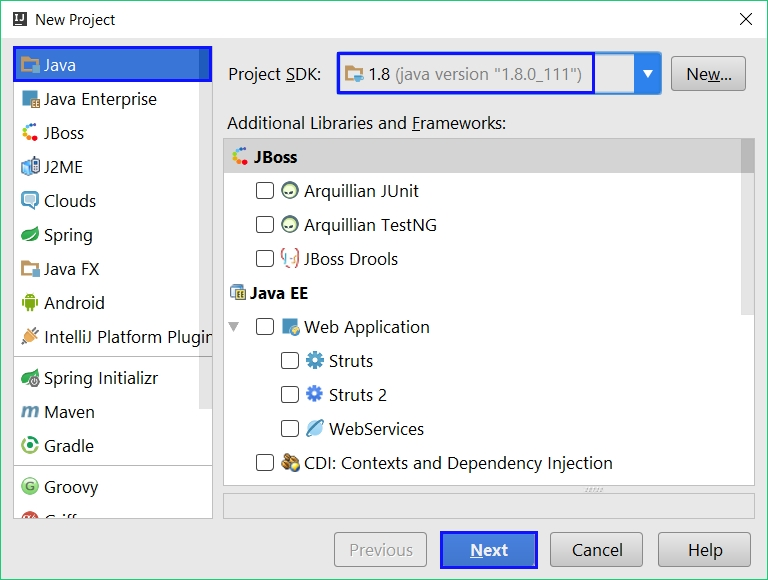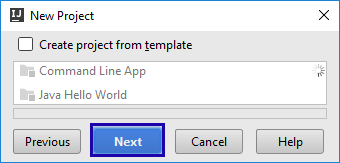Give an appropriate name for the project, for example "SimpleCalculations" and also where to be stored our project, and after that we click [Finish]: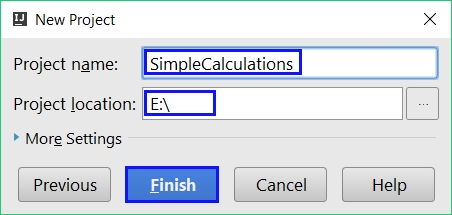Now we have empty IntelliJ IDEA Project (without any Java classes in it which have to be in the src folder):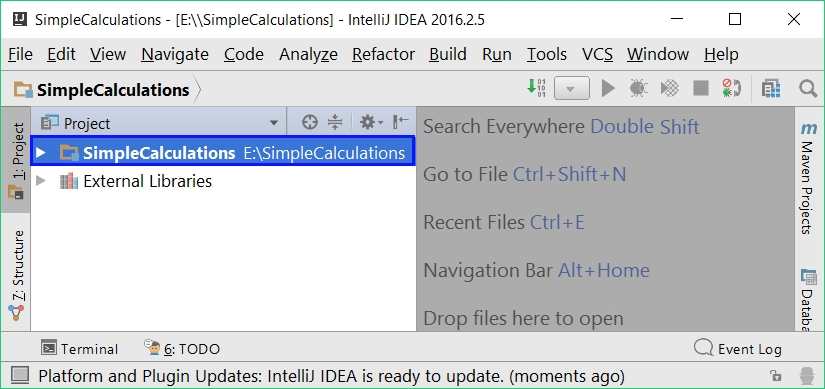The purpose of this project is to add in it .java class per problem from the exercises.

### Exercise: Calculating the area of the square

The first task from this topic is the following: write a console program that read as input data an integer a and calculates the area of a square with side a. The task is trivial and easy: read as input data a number from the console, then multiply it by itself and print the obtained result as an output on the console.

#### Hints and guidelines

We create a new Java class in the existing IntelliJ IDEA project. On the src folder, right-click with the mouse. Choose [New] → [Java Class]: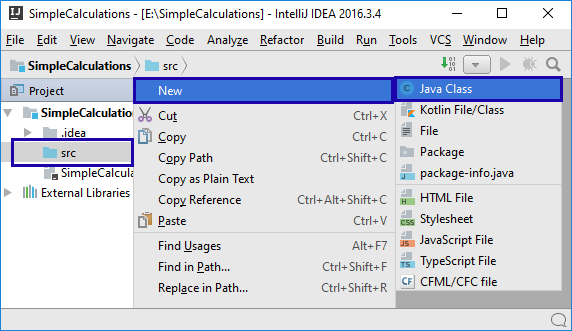A dialogue window will open for choosing а name to the Java class, for example, “SquareArea”: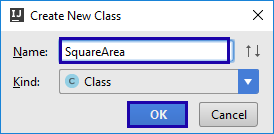We already have a Project with Java class in it. What remains is to write the code for solving this problem. For this purpose, we write in our Java class a Main method(as in the picture below). In the body of the Main method Main(string[] args) we write the following code between the opening and closing curly brackets: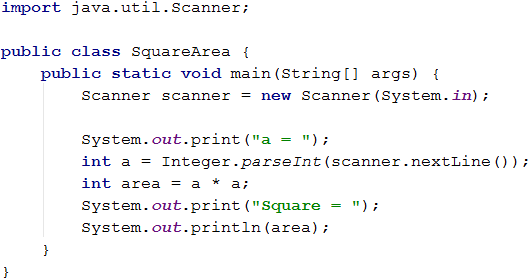As we can see in the code above, we read as input data an integer and assign it to a variable a (line int a = Integer.parseInt(scanner.nextLine())), afterwards we calculate the area (line int area = a * a) and finally prints the value of the variable area on the console. We start the program with [Ctrl+Shft+F10] and we test it with different input values: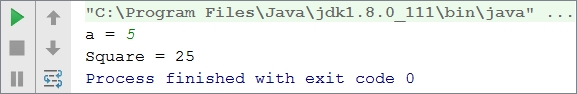#### Testing in the Judge system

Test your solution here: https://judge.softuni.org/Contests/Practice/Index/649#0. You have to get 100 points (completely correct solution):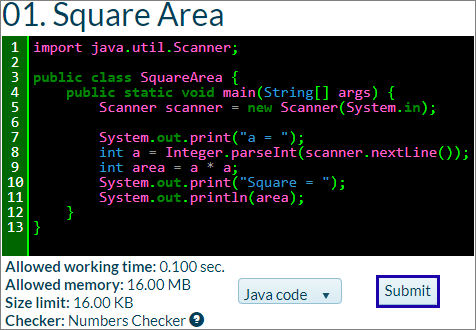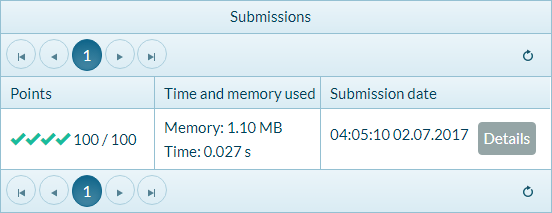### Exercise: Inches to centimeters

Write a program that reads a number from the console (not necessarily an integer) and converts the number from inches to centimeters. For this purpose multiply the inches by 2.54 (because one inch = 2.54 centimeters).

#### Hints and guidelines

First, we create a new Java class in the project “SimpleCalculations”. We click on the folder src and choose [New] → [Java Class]: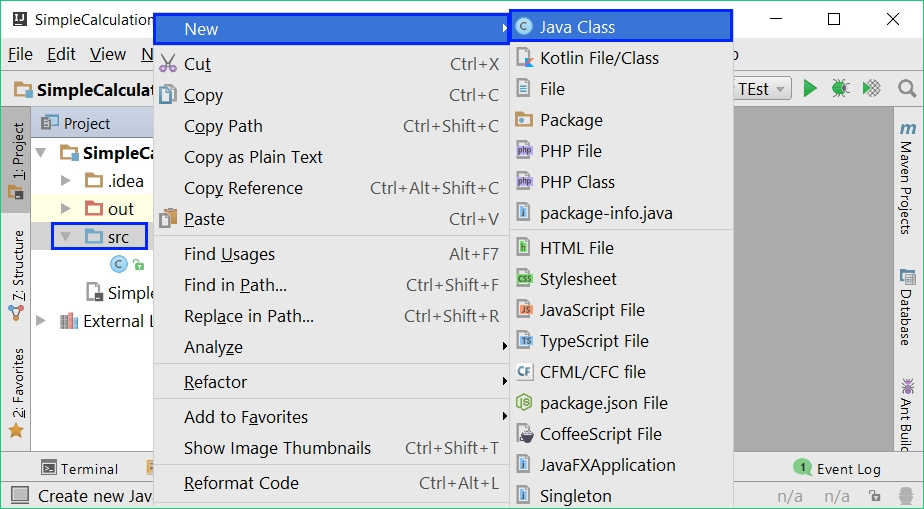A dialogue window appears in which we write the file’s name. In our case this is "InchesToCentimeters" → [OK]: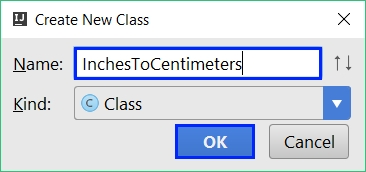Next, we have to write the program code: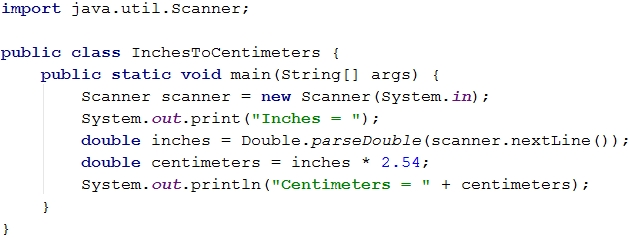We start the program with the right click on the window of the current program. We choose [Run InchesToCentim...main()] or we press [Ctrl+Shift+F10]. This shortcut starts the program in the current Java class (the one in which we wrote last):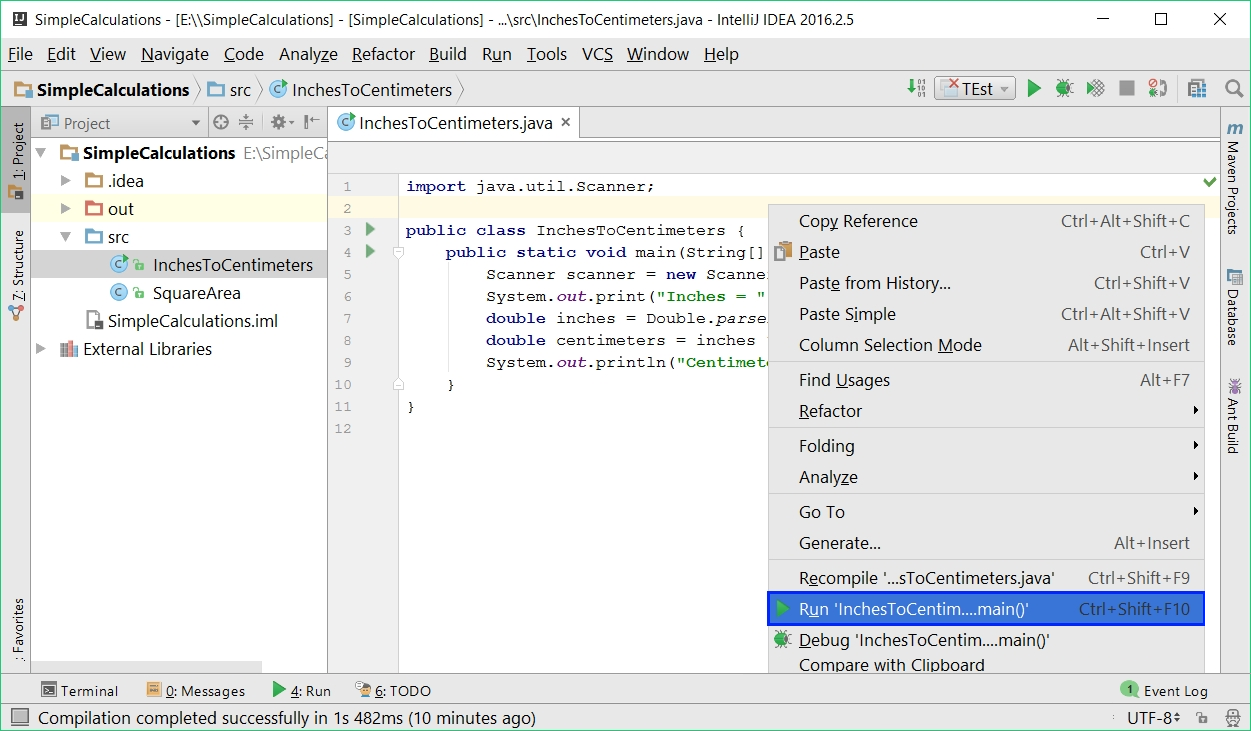We obtain the following result: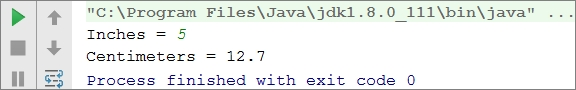Now let's switch to the previous program/task ("Square Area"). This is done by double-clicking on the file SquareArea.java from the folder src in the project [SimpleCalculations]: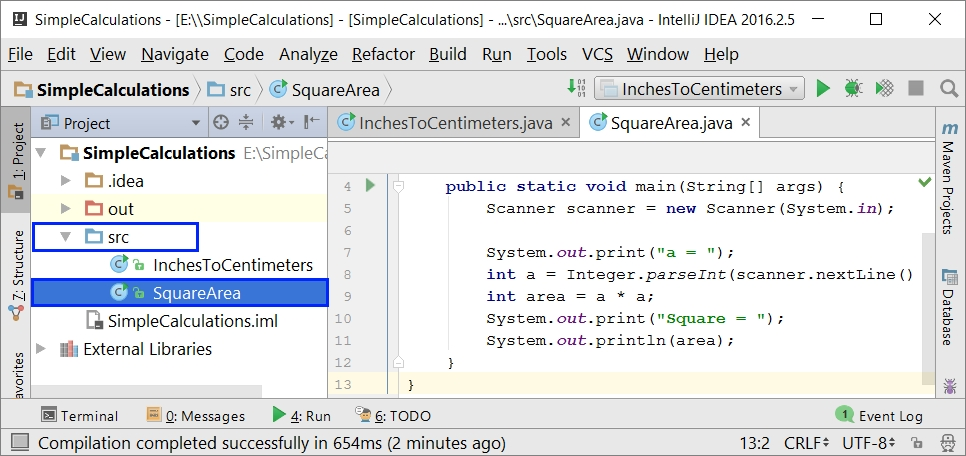If we use [Shift+F10] or press one of the two green arrows (located at the top right and bottom left of the IntelliJ IDEA window)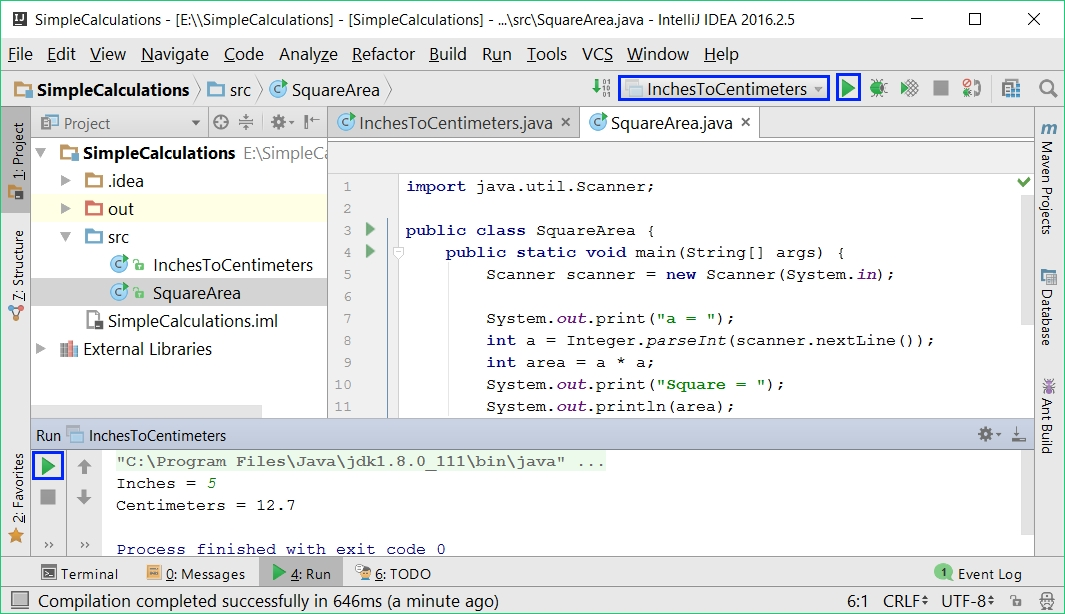the last compiled program will be executed (InchesToCentimeters). We can see it as a name in the upper right corner of the window in front of one of the two green arrows: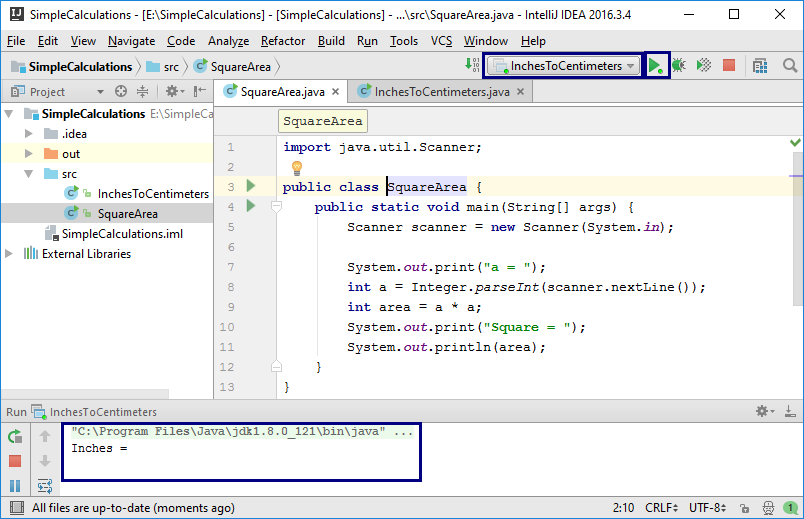Switching between projects is an easy task, isn't it? Choose the file with the source code of the program, double click on it and when it starts, the program from the current file runs.

Let's test with floating-point numbers, for example, with 2.5: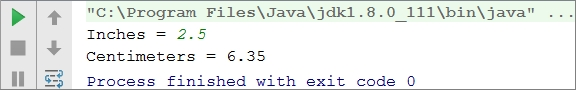Depending on the regional settings of the operating system, it is possible to use decimal point (US settings) instead of decimal comma (BG settings).

If the program expects a decimal point and instead you enter a number with a decimal comma or the opposite (to enter a decimal point, when a decimal comma is expected), the following error will be produced: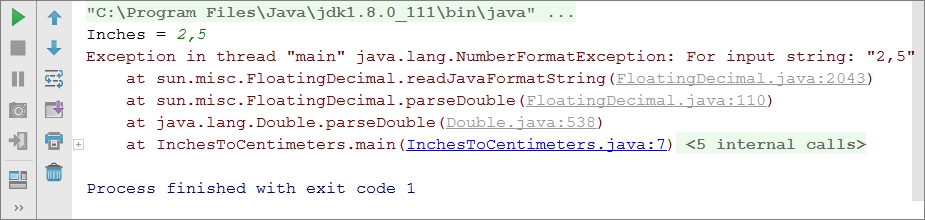It is recommended to change the settings of your computer, to use a decimal point: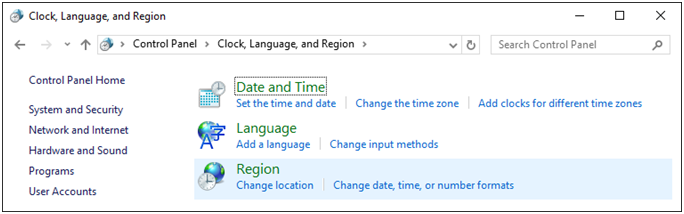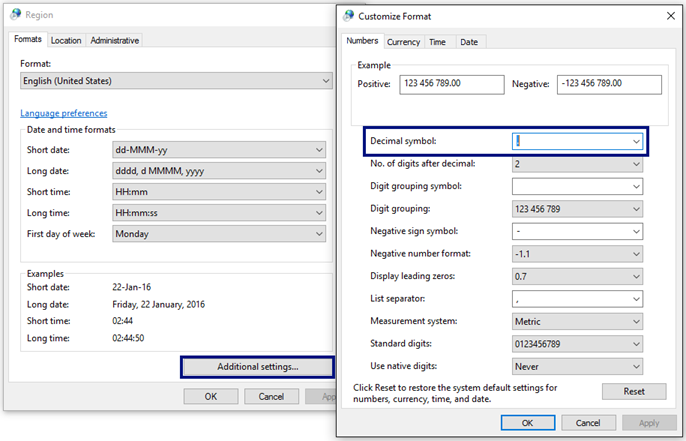#### Testing in the Judge system

The solution should be accepted as a completely correct one: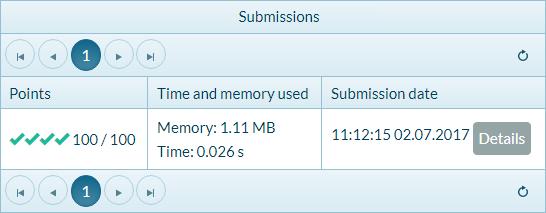### Exercise: Greeting by name

Write a program that reads from the console a person's name and prints Hello, <name>!, where <name> is the name entered earlier.

#### Hints and guidelines

First, we create a new Java class with the name “Greeting” in the project “SimpleCalculations”: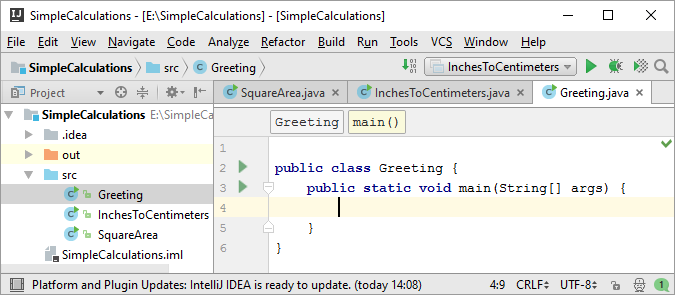Next, we have to write the code of the program. If you have any difficulties, you can use the code from the example below: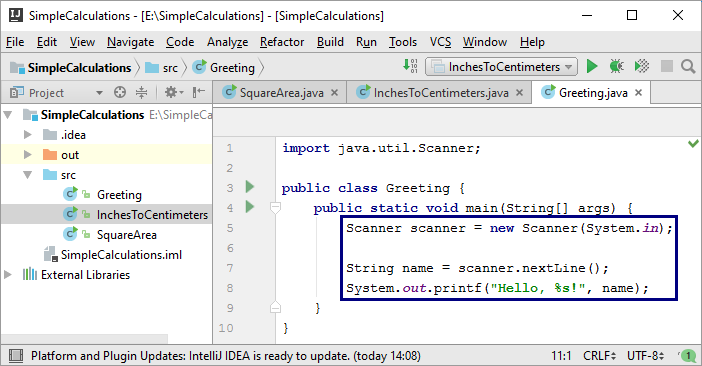Run the program with [Ctrl+Shift+F10] and test if it works: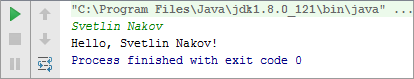### Exercise: Concatenating text and numbers

Write a Java program, that reads from the console a first name, last name, age, and city, and prints a message of the following kind: You are <firstName> <lastName>, a <age>-years old person from <town>..

#### Hints and guidelines

We add to the existing IntelliJ IDEA Project one more Java class with the name "ConcatenateData". We write the code, which reads the input from the console: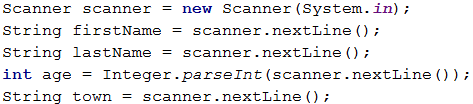You should write the code that prints the message described in the requirements.In the picture above, the code is consciously blurred on purpose, in order for you to think of a way to finish it yourself.

Next, test the solution locally using [Ctrl+Shift+F10] and by entering sample input data.

### Exercise: Trapezoid area

Write a program that reads three numbers from the console b1, b2 and h and calculates the area of a trapezoid with bases b1 and b2 and height h. The formula for the area of trapezoid is (b1 + b2) * h / 2.

Тhe figure below shows a trapezoid with bases 8 and 13 and height 7. It has an area (8 + 13) * 7 / 2 = 73.5.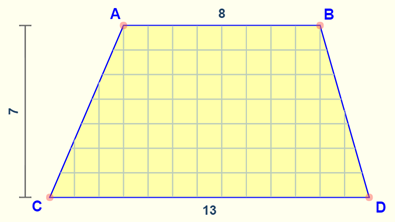#### Hints and guidelines

Again, we have to add to the existing IntelliJ IDEA Project another Java class with the name "TrapezoidArea" and to write the code that reads the input from the console, then calculates the area of the trapezoid, and prints it as an output on the console: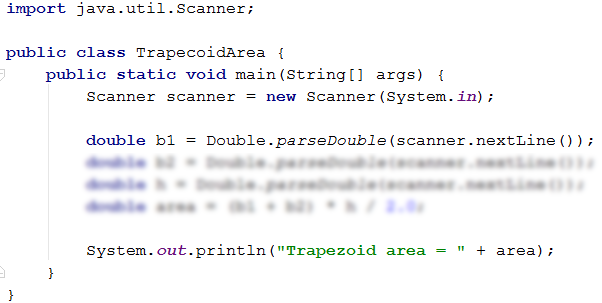The code on the picture is consciously blurred for you to give a thought and finish yourself.

Test your solution locally using [Ctrl+Shift+F10] and by entering sample input data.

### Exercise: Circle area and perimeter

Write a program that reads from the console a number r r then calculates and prints the area and perimeter of the circle/round with radius r.

#### Sample input and output

Input Output
3 Area = 28.2743338823081
Perimeter = 18.8495559215388
4.5 Area = 63.6172512351933
Perimeter = 28.2743338823081

#### Hints and guidelines

For the calculations, you can use the following formulas:

• Area = Math.PI * r * r.
• Perimeter = 2 * Math.PI * r.

### Exercise: Rectangle area

A rectangle is defined by the coordinates at two of its opposite angles (x1, y1) – (x2, y2). Calculate its area and perimeter. The input is read from the console. The numbers x1, y1, x2 и y2 are given one per line. The output is printed on the console and it has to contain two lines, each with one number – the area and the perimeter.Input Output
60
20
10
50
1500
160
30
40
70
-10
2000
180
600.25
500.75
100.50
-200.5
350449.6875
2402

### Exercise: Triangle area

Write a program that reads from the console a side and height of a triangle and calculates its area. Use the formula for triangle area: area = a * h / 2. Round the result to 2 digits after the decimal point using Math.round(area, 2).

#### Sample input and output

Input Output
20
30
Triangle area = 300
15
35
Triangle area = 262.5
7.75
8.45
Triangle area = 32.74
1.23456
4.56789
Triangle area = 2.82

### Exercise: Converter – from °C (Celsius) to °F (Fahrenheit)

Write a program that reads degrees on Celsius scale (°C) and converts them to degrees on Fahrenheit scale (°F). Look on the Internet for a proper formula, to do the calculations. Round the result to 2 digits after the decimal point. Here are a few sample data:

Input Output
25 77
0 32
-5.5 22.1
32.3 90.14

### Exercise: Converter – from radians to degrees

Write a program, that reads an angle in radians (rad) and converts it to degrees) (deg). Look for a proper formula on the Internet. The number π in Java programs is available through Math.PI. Round the result to the nearest integer using the method Math.round(…).

Input Output
3.1416 180
6.2832 360
0.7854 45
0.5236 30

### Exercise: Converter – USD to BGN

Write a program for conversion of US dollars (USD) into Bulgarian levs (BGN). Round the result 2 digits after the decimal point. Use a fixed rate between a dollar and levs: 1 USD = 1.79549 BGN.

Input Output
20 35.91 BGN
100 179.55 BGN
12.5 22.44 BGN

### Exercise: * Currency converter

Write a program for conversion of money from one currency into another. It has to support the following currencies: BGN, USD, EUR, GBP. Use the following fixed currency rates:

Exchange rate USD EUR GBP
1 BGN 1.79549 1.95583 2.53405

The input consists of three lines - the first is a sum for conversion, the second is the input currency, and the third is output currency. The output is one number – the converted value according to the above exchange rates, rounded 2 digits after the decimal point.

Input Input
20
USD
BGN
35.91 BGN
100
BGN
EUR
51.13 EUR
12.35
EUR
GBP
9.53 GBP
150.35
USD
EUR
138.02 EUR

### Exercise: ** Date calculations – 1000 days on the Earth

Write a program that reads from the console a birth date in format dd-MM-yyyy then calculates the date on which 1000 days are turned since this birth date, and prints the output in the same format.

#### Sample input and output

Input Output
25-02-1995 21-11-1997
07-11-2003 03-08-2006
30-12-2002 25-09-2005
01-01-2012 27-09-2014
14-06-1980 11-03-1983

#### Hints and guidelines

• Look for information about the types Date, Calendar and SimpleDateFormat in Java and in particular look at the methods Calendar.setTime(date), Calendar.add(countDays) and SimpleDateFormat.format(date). With their help, you can solve the problem without the need to calculate days, months, and leap years.
• Don't print anything additional on the console except for the wanted date!